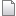# Guile Scheme Modules

I have written some Guile Scheme functions and macros that I have found useful, and have packaged them up as Guile modules. (More Guile modules and scripts I have written can be found here.)

## Introduction

While not formally documented, most functions have comments regarding their usage. Some useful functions and macros include:

• The macros `when` and `unless`.
• Anaphoric macros as found in Paul Graham's On Lisp book, like `aif`, `aand`, and `acond`.
• Macros like `compose-chain`, `let-nth`, and `slot-mod!`.

You can browse the sources here.

You can download the source code from the table of attachments below.

## compose-chain Macro

The `compose-chain` macro lets you unravel complex compositions into a more linear and legible form. It has the following form:

```  (compose-chain (var)
expr1
expr2 ...)
```
First, expr1 is evaluated and the result bound to var for expr2 to use. The result of expr2 is then bound to var for the next expression to use. The result of the evaluation of the final expression is returned as the result of `compose-chain`.

For example, the following expression:

```  (enumerate* (shape-square
(row (row (make <pin> #:thickness 10) rows 0 row-pitch)
pins-per-row pin-pitch 0)
0)
1)
```
can be rewritten like this:
```  (compose-chain (p)
(make <pin> #:thickness 10)
(row p rows 0 row-pitch)
(row p pins-per-row pin-pitch 0)
(shape-square p 0)
(enumerate* p 1))
```
which is itself equivalent to:
```  (let* ((p (make <pin> #:thickness 10))
(p (row p rows 0 row-pitch))
(p (row p pins-per-row pin-pitch 0))
(p (shape-square p 0)))
(enumerate* p 1))
```

## let-nth Macro

The `let-nth` macro is useful for binding variables to values taken sequentially from a list. It has the following form:

```  (let-nth ((var expr) ...)
body ...)
```
`let-nth` is just like Scheme's `let*`, except that the environment for evaluating each expr has bound within it a function called `nth`. The `nth` function defined for the first expr is equivalent to `(lambda (x) (list-ref x 0))`; i.e., it extracts the first element of a list. The `nth` defined for the second expr is equivalent to `(lambda (x) (list-ref x 1))`; i.e., it extracts the second element of a list. And so on.

While I've found `let-nth` useful in a variety of situations, lately I've used it to extract position-based command line arguments from within Guile scripts. For example, here's a snippet from a script where `parameters` is a list of command line arguments, and where the first argument is a description, the second argument is a pin count, etc.:

```  (let-nth ((description (nth parameters))
(pin-count (string->number (nth parameters)))
(pin-width (evaled-string->cmil options (nth parameters)))
(pin-length (evaled-string->cmil options (nth parameters)))
(pin-offset (evaled-string->cmil options (nth parameters)))
(component-width (evaled-string->cmil options (nth parameters)))
(body-width (evaled-string->cmil options (nth parameters))))
...)
```

## slot-mod! Macro

The `slot-mod!` macro is like `slot-set!`, except that you can reference the previous value of the slot using the `<>` symbol. For example, assuming that an object, `obj`, has a slot named `x`, you can replace the current value of slot `x` with its square with the following expression:

```  (slot-mod! obj 'x (* <> <>))
```
This is equivalent to the following expression:
```  (slot-set! obj 'x (* (slot-ref obj 'x) (slot-ref obj 'x)))
```

## Installation

To install the Guile modules:

• `tar -xzf octw-guile-general-modules-1.0.tar.gz`
• Change the `INSTALL_DIR` to point to the directory in which you would like to install the modules.
4. Run `make` to install the modules.
 Attachment Size Date Commentgz octw-guile-general-modules-1.0.tar.gz 15.7 K 09 Sep 2008 - 22:01 General Guile Library 1.0